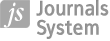RESEARCH PAPER
The Golden Rules of Capital Accumulation in the Gravity Model of Economic Growth

More details
Hide details
Publication date: 2015-06-30

GNPJE 2015;277(3):27–47

KEYWORDS
JEL CLASSIFICATION CODES
ABSTRACT
The paper seeks to determine the so-called golden rules of capital accumulation in a model of economic growth known as the gravity model. This model combines Solow’s neo-classical model of economic growth with what is defined as the gravity effect. The authors consider two variants of the gravity model. The first variant seeks such a level of investment rates that would maximize the geometric average of consumption per employee in all economies, under the assumption of a long-term equilibrium. The second variant seeks such a level of investment rates that would maximize long-term consumption per employee in each economy. The research shows that, in both variants, optimum investment rates depend on the elasticity of production with regard to capital and on the gravity effect, the authors say. In the second variant, the optimum investment rates additionally depend on the number of economies included in the model. If the number of economies subject to the gravity effect increases, investment rates decrease in each economy, the authors say. In both variants, the gravity effect peters out and is eventually reduced to zero, but tends to have the same form as the original golden rules of capital accumulation posited by Edmund Phelps, the authors argue. They add that the golden rules of accumulation determined in the article are a generalization of Phelps’ original golden rules of capital accumulation under the assumption of the existence of a gravity effect.
RELATED ARTICLE
 eISSN: 2300-5238